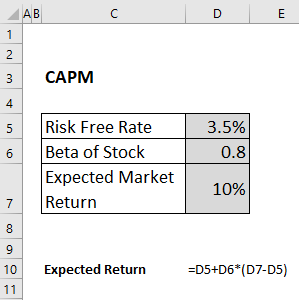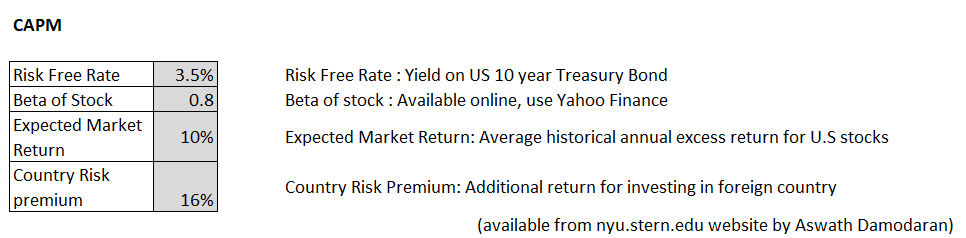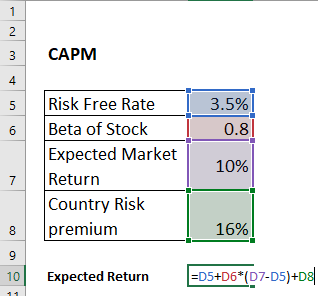### How to Calculate CAPM?

CAPM or Capital Asset Pricing Model helps to calculate the cost of equity for an investment. Using the CAPM formula we can find the expected return for an asset. It can further be used for financial ratio like – Sharpe Ratio and others.

### CAPM Formula with its components

Er = Rf + [Bi x (Rm – Rrf)]

Step 1: Input the values which act as input for the CAPM Model. The details of the input will be available online as mentioned in the sources.Step 2. Calculate Expected Return using the CAPM Formula.

Expected Return: Risk Free Rate + Beta *(Expected Market Return – Risk Free rate)Expected return = 8,7%

### CAPM with Country Risk premium(CRP) – For Foreign Investments

Country Risk premium is the addition return that an investor can expect if he is investing in a foreign company.

When to use CRP?

• If the investment is done in foreign land, overseas investment
• If there is higher political and geographical risk associated with this risk
• CRP for developing / turbulent countries is higher than developed countries. The higher the risk of a country the higher the value of CRP.Formula:

Expected Return: Risk Free Rate + Beta *(Expected Market Return – Risk Free rate) + Country Risk PremiumExpected return = 24,7%

## Template

```Further reading: